Deutsche Version● 1 - Standing waves (acoustic resonance) on ideal strings
Standing waves are stationary waves

 A standing wave is the vibration state of a stable system. An example of this is the vibrating string of a musical instrument, or the column of air in an organ pipe. These waves have a wave-like look, but nothing is moving. The string is clamped between two fixed ends with length L; which is called a node also known as a zero crossing. The location directly between two nodes is called an antinode. Shown below are the natural vibrations of an idealized string, which is assumed to be perfectly flexible. The stiffness is assumed to be zero. The frequencies of the vibrations form harmonics, also called partials. c = speed of sound. L = length of the string. The fundamental wave is known as the first harmonic.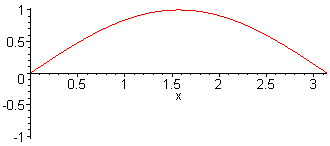1st harm.   L = λ/2 = π        f1 = c / (2·L)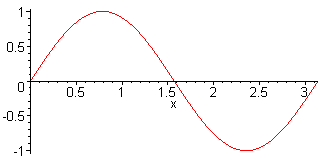2nd harm.   L = λ        f2 = 2·f1 = c / L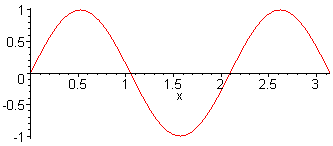3rd harm.   L = 3 λ / 2       f3 = 3·f1 = 3·c / (2·L)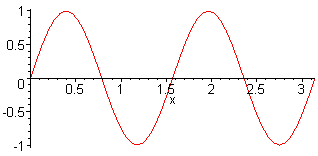4th harm.   L = 2 λ        f4 = 4·f1 = 4·c / (2·L)Note: Note: The distance between two nodes (knots) or two maxima (antinodes) is λ/2. The distance between a node (maximum) and an antinode (minimum) is λ/4.

 Note, because there is often confusion: An antinode in sound pressure (pressure antinode) is at the same time a node in the displacement of the air particles (particle displacement node). It is mostly forgotten to tell the viewer of the figures what is shown: sound pressure derivations or motion of particle displacement. That's a difference.Druck = pressure and Frequenz = frequency  1st harm.   L = λ / 2 = π     f1 = c / (2 · L)  2nd harm.   L = λ                  f2 = 2 · f1 = c / L  3rd harm.   L = 3 λ / 2         f3 = 3 · f1 = 3 c / (2 · L)   Open tube

 Oscillations of a column of air of an open tube (flute). At the open end theoretically there must be a sound pressure node, if one leaves aside the end correction.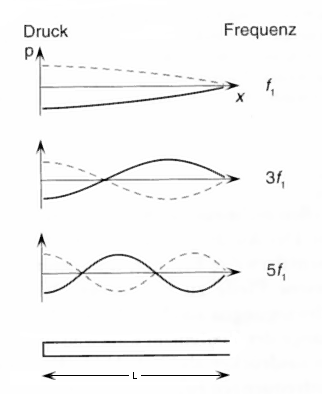Druck = pressure and Frequenz = frequency  1st harm.   L = λ / 4 = π · 1 / 4    f1 = c / (4 · L)  3rd harm.   L = 3 λ / 4                  f3 = 3 · f1 = 3 c / (4 · L)   5th harm.   L = 5 λ / 4                   f5 = 5 · f1 = 5 c / (4 · L)   Left single-edge closed tube

 Oscillations of an air column of a single-edge closed tube (flute); also stopped pipe (gedackt). At the closed end theoretically there must be an antinode of the sound pressure.

 There are misunderstandigs of waves in air, because sinusoidal patterns can indicate the nodes and antinodes of the displacement of the sound, the particle velocity of the sound, and the sound pressure or the sound density. Sound engineers using microphones are only interested in the behavior of the sound pressure deviations. Notice: A pressure node corresponds to a displacement antinode!

3 - Room modes (standing waves) between sonically hard parallel walls

Where do I set up my speakers? Where is the best listening spot? Where to go with the acoustic treatment?
The following fundamentals for the acoustics of low frequency sound waves in rooms you should know.
This applies to the recording room as well as for the playback room.

 The room resonances formed between the boundary surfaces of a room, are called "standing waves" or room modes, or modes. They arise if a multiple of half the wavelength (λ/2) fits between the boundary surfaces of an area. Therefore, one need not necessarily parallel walls. Sound technicians are interested in the behavior of the sound pressure, because by its effect our eardrums and the microphone diaphragms are moved, see: Effect and cause.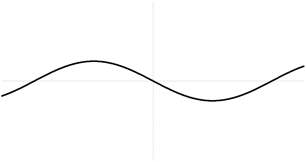A standing wave as sound pressure distribution:
3rd harmonic (2nd overtone)
− 3 nodes and 4 antinodes

Room modes as sound pressure distribution

Calculation of the three modes (eigenmodes) - resonances of rectangular rooms

An eigenmode (eigentone) is the European name for a standing wave.Note: In practice only low frequencies as sound pressure below 300 Hz are considered as room modes. Higher modal frequencies become less important, because their disturbing effect is masked by other acoustic effects. On the walls there are formed by the modes always sound pressure maxima - that are sound pressure antinodes (or particle velocity minima = nodes).

 By the following formula standing waves that are room resonances (modes), which are built between the reverberant boundary surfaces of a rectangular room, can be determined in their frequency. On a sound-reflecting wall (boundary) the absorption coefficient is α = 0.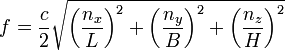Where: f = frequency of the mode in Hz c = speed of sound 343 m/s at 20 °C nx = numbers of natural oscillations (room length) (1, 2, 3, ...) ny = numbers of natural oscillations (room width) (1, 2, 3, ...) nz = numbers of natural oscillations (room height) (1, 2, 3, ...) L, B, H = length, width and height of the room in meters A standing wave 1st order of the fundamental frequency = f1 occurs, when half the wavelength of the excitation frequency fits between the sonically hard boundary surfaces. For the axial mode we get the lowest frequency : f1 = c / (2 · L) Where: c = speed of sound343 m/s at 20 °C f1 = frequency in Hz of the axial mode L = longest distance in meters of the boundary surfaces. The standing waves of higher order are calculated from the integer multiples of the 1st order mode. f2 = 2 · f1 f3 = 3 · f1 f4 = 4 · f1Antinodes pressure maximum Nodes zero crossing Wavelength Frequency Harmonics Overtones 2 1 λ = 2 × L f1 = 1 × c / (2 × L) 1st harmonic fundamental 3 2 λ = L f1 = 2 × c /(2 × L) 2nd harmonic 1st overtone 4 3 λ = (2 × L) / 3 f1 = 3 × c / (2 × L) 3rd harmonic 2nd overtone k + 1 k λ = (2 × L) / k f1 = k × c / (2 × L) k harmonic (k − 1) overtone

 k = 1, 2, 3, ...

 The sound pressure is actually a longitudinal wave a), in contrast to this more presentable transverse wave b).

 Direction of oscillation                              Direction of propagation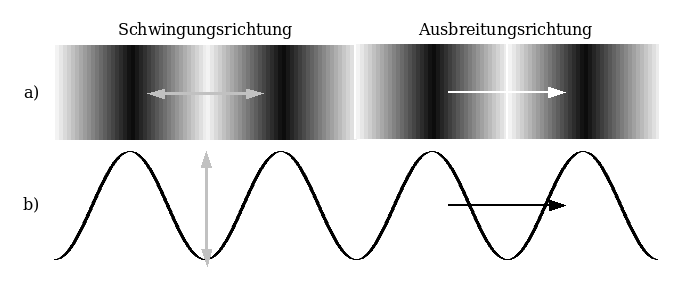Standing wave modes as sound pressure distribution between the walls. Wave with two open ends.1st harm.   L = λ/2 = π        f1 = c / (2·L)2nd harm.   L = λ        f2 = 2·f1 = c / L3rd harm.   L = 3 λ / 2    f3 = 3·f1 = 3·c / (2·L)4th harm.   L = 2 λ       f4 = 4·f1 = 4·c / (2·L)

 Here, the sound pressure of a sine wave with harmonics is presented between two totally reflecting walls which in reality is a longitudinal wave. It is important that in the case of resonance there is always a maximum sound pressure on the walls. In many figures this is wrongly shown as a vibration of a string, which has always a fixed node (knot) at each end, as in the following example:

 This is not the representation of sound pressure vibrations between walls in a small room. These are vibrations of a string (displacement amplitudes).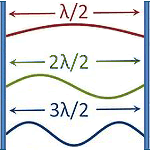A typical wrong figure with  nodes at the walls.  Showing the air displace-   ment was not desired.

 A string is clamped between two "fixed ends". At the ends will be a wave node. A wave between two hard walls is considered as a sound pressure with "loose ends". Therefore on the sides of the wall there appears as sound pressure a wave maximum or an antinode.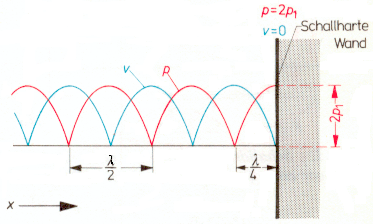Sonically hard wall

 Spatial distribution of sound pressure p (red) and particle velocity v (blue) in a schematized standing wave with total reflection from a sonically hard wall. At a distance of λ / 4 from the wall the sound pressure is p = 0. Since the absorption effect increases in a material with the sound velocity, the absorber should be effective in the velocity maximum at the distance of λ / 4 from the wall or should have an appropriate density. Much easier measurable is the sound pressure minimum, at the exact spot of velocity maximum.

 Here is a nice animation of a standing wave - Explanation by superposition with the reflected wave. This setting must be: "Reflection from a free end". http://www.walter-fendt.de/ph14e/stwaverefl.htmConclusion: The vibrations of sound pressure between parallel walls in standing waves as room modes are looking much different with "open ends" at the walls (antinodes) than the vibrations of strings between two "fixed ends" (nodes). Remember: At reflective walls (boundaries) we find always the maximum sound pressure, a so called wave antinode. This is also the principle of the boundary layer microphones.

 The frequency at which the first (lowest) room mode occurs, is calculated using the following formula: Frequency = speed of sound c / (longest room dimension × 2)

 Comparison of the reflections in an echo chamber and an extremely highly damped room.Sound pressure reflections (modes) at sonically hard walls, like in an echo chamber (wet).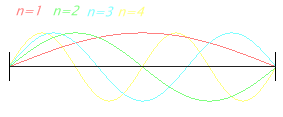Sound pressure reflections (modes) at sonically soft walls, like in a reflection free room (dry).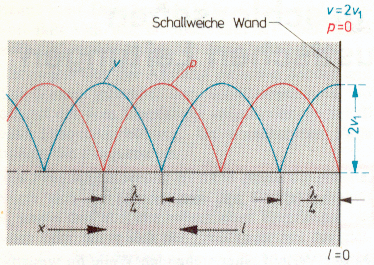Sonically soft wall

 Spatial distribution of sound pressure p (red) and particle velocity v (blue) in a schematized standing wave with total reflection from a sonically soft wall. At a distance of λ / 4 from the wall the sound pressure is p = maximum.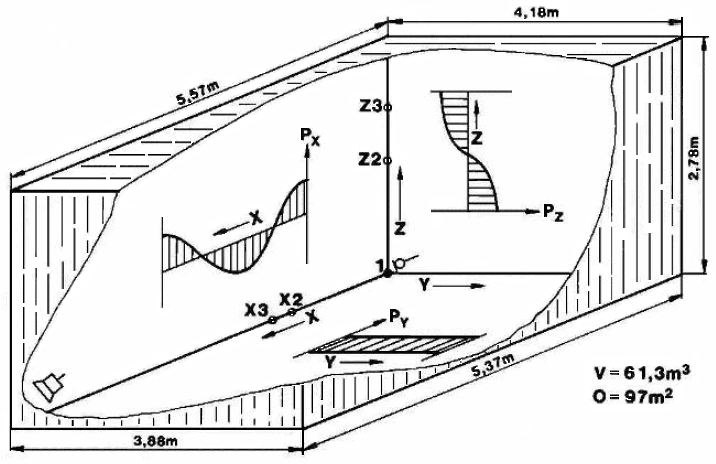A typical room (control room) with axial modes and sound pressure anti nodes at the walls

 Room Eigenmodes Calculator − Jörg Hunecke This calculator determines the room eigenmodes with the 20 lowest eigenfrequencies for rectangular rooms and presents them in ascending order.

The Pressure Chamber Effect

 Even below the lowest room mode low frequencies are reproducible. One uses the pressure chamber effect in a with hard boundary surfaces equipped small room, where the room must be sealed pressure-tight. The "dB Drag Racing" fans need that for their car loudness competitions.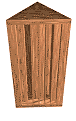RPG Triffusor

 Absorption - Reflection - Diffusion - Combination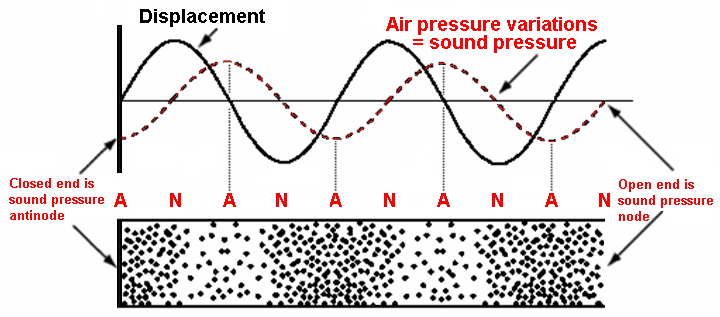Notice: At a hard reflecting wall (closed end) there is a sound pressure antinode but at the same time there is a particle displacement node. When one speaks of standing waves, it must be expressed clearly that we mean amplitudes of the acoustic pressure changes that move our eardrums. That is mostly forgotten. The sound pressure derivtions of air are oscillating with antinodes and nodes - at the same time the displacement of air particles are oscillating with nodes and antinodes. The relationship between the maximum pressure change Δ pm and the maximum displacement amplitude of the air particles ξm is: Δ pm = (v ρ ω) ξm.

Amplitudes of the Soundfield Quantities of a Plane Wave

 Distinguish the amplitudes of particle displacement, sound pressure, sound velocity, and pressure gradient. Sound pressure antinode is particle displacement node of the air particles. Particle displacement antinode of the air particles is sound pressure node.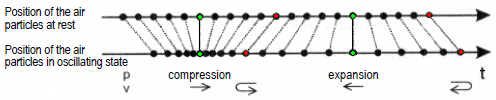At rest, a green air particle has the same position as in the oscillation condition. The displacement of air particles is going through a zero crossing while the sound pressure amplitude with p maximum (condensation, compression) or a minimum (rarefaction, expension) shows. At rest, a red air particle is at the maximum or minimum of the amplitude of the same time there is a zero crossing of the sound pressure wave. Thus, there the sound pressure is zero; ie the normal static air pressure is present alone.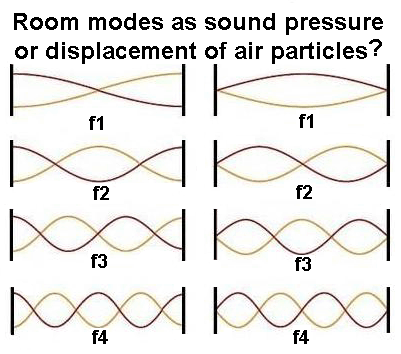In graphic representations of standing waves (room modes) between two walls, usually there is not specified whether there is shown the sound pressure as the pressure variation in the air, or the displacement of the air. However, this clarification is really necessary. The figures above show the important difference. Left = sound pressure (antinodes) and right = displacement (nodes).   Notice: The effect of standing waves reports our hearing, but the sound pressure deviations (fluctuations, variations) move effectively our eardrums.